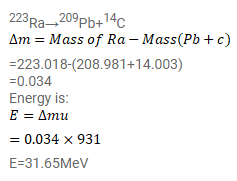# Find the energy liberated are as follows:

Question:

Find the energy liberated are as follows:

223Ra209Pb+14C

The atomic masses needed are as follows:

$\begin{array}{lll}{ }^{223} \mathrm{Ra} & { }^{209} \mathrm{~Pb} & { }^{14} \mathrm{C} \\ 223.018 \mathrm{u} & 208.981 \mathrm{u} & 14.003 \mathrm{u}\end{array}$

Solution: